# E-PolyLearning

 31. Choose the figure which is different from the rest.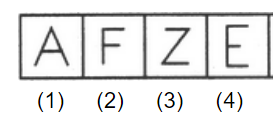a. 1 b. 2 c. 3 d. 4

 32. Choose the figure which is different from the rest.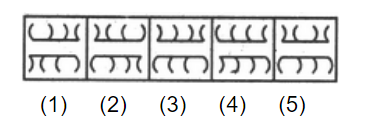a. 1 b. 2 c. both 3 and 4 d. 5
 33. Choose the figure which is different from the rest.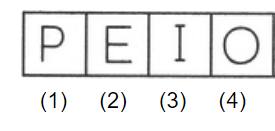a. 1 b. 2 c. 3 d. 4
 34. Choose the figure which is different from the rest.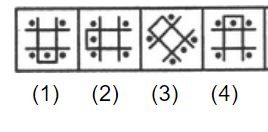a. 1 b. 2 c. 3 d. 4
 35. Choose the figure which is different from the rest.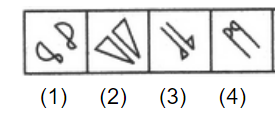a. 1 b. 2 c. 3 d. 4